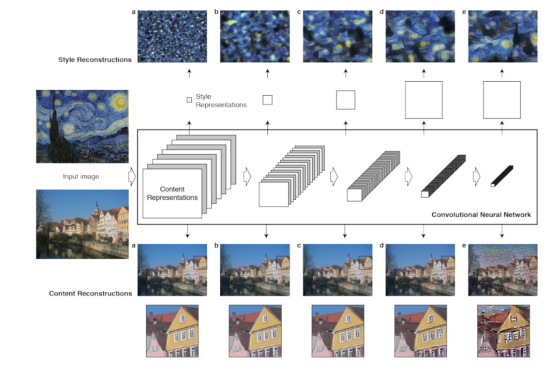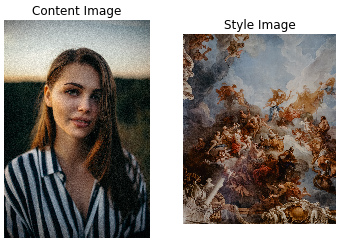# 使用TensorFlow Hub进行神经风格迁移• 神经风格迁移的描述
• 神经风格迁移如何工作
• 风格迁移的代码实现

### 什么是神经风格迁移

NST是一种图像风格化方法，它是一种使用输入参考图像来提供从输入图像导出的风格变化的输出图像的过程。

Leon A Gatys等人在论文 “艺术风格的神经算法”中（https://arxiv.org/pdf/1508.06576.pdf） 介绍了NST技术。### 如何运作

CNN生成的输入图像的空间表示形式捕获图像的风格和内容统计信息。NST将提取的风格和内容组合到生成的输出图像中。

CNN层结构内的中间层中的激活函数提供了捕获输入图像的内容和风格统计信息的功能。

CNN图层在卷积操作之后输出特征图，该卷积操作涉及在输入图像上有一个滤波器滑动。图像的内容实际上在每个图层的生成的特征图中。### 编程TensorFlow Hub提供了一套可重用的机器学习组件，例如数据集，权重，模型等。

• TensorFlow：一个用于实施，训练和部署机器学习模型的开源平台。
• Matplotlib：用于在Python中创建可视化图的工具，例如图表，图形等
• numpy：启用数组数据结构的几种数学计算和运算。
• TensorFlow Hub：可重用的机器学习组件库，例如模型，数据集等

import tensorflow as tf
import matplotlib.pyplot as plt
import numpy as np
import PIL.Image
import tensorflow_hub as hub

content_path = 'images/human.jpg'
style_path = 'images/painting.jpg'
plt.subplot(1, 2, 1)
plt.title('Content Image')
plt.axis('off')
imshow(content_image)
plt.subplot(1, 2, 2)
plt.title('Style Image')
plt.axis('off')
imshow(style_image)def image_to_tensor(path_to_img):
img = tf.image.decode_image(img, channels=3, dtype=tf.float32)

# Resize the image to specific dimensions
img = tf.image.resize(img, [720, 512])
img = img[tf.newaxis, :]
return img

def tensor_to_image(tensor):
tensor = tensor*255
tensor = np.array(tensor, dtype=np.uint8)
tensor = tensor
plt.figure(figsize=(20,10))
plt.axis('off')
return plt.imshow(tensor)

• 查看内容和参考风格图像
• 创建一个函数将图像转换为张量，并将张量转换为图像

content_image_tensor = image_to_tensor(content_path)
style_image_tensor = image_to_tensor(style_path)
combined_result = hub_module(tf.constant(content_image_tensor), tf.constant(style_image_tensor))
tensor_to_image(combined_result)### 结论

https://github.com/RichmondAlake/tensorflow_2_tutorials/blob/master/03_neural_style_transfer.ipynb

http://panchuang.net/

sklearn机器学习中文官方文档：
http://sklearn123.com/

http://docs.panchuang.net/